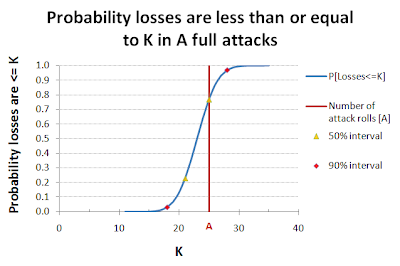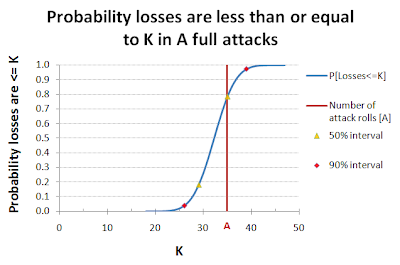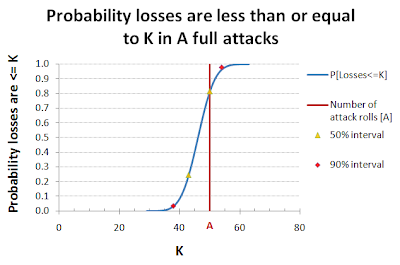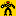## 25 July 2011

### Taking a RISK - the distribution of armies lost

I came across the following question at boardgames.stackexchange.com:
How can I estimate my chances to win a Risk battle?Elliot Avedon Virtual Museum of Games, Courtesy Canadian Museum of Civilization.
Now there is already plenty of material on the web about probability in the popular boardgame RISK*, but maybe I can add just a little bit more.

Most people already know that, given the choice, the Attacker should always 3 dice and the Defender should always roll 2, since this always gives the best results (a Nash equilibrium). Since it's always the Attackers choice to roll an attack or not, the relevant question seem to be "How many armies [X] will the Attacker lose if they make [N] attack rolls?"Using some probabilities from a RISK FAQ for the probabilities of losses, I calculated the average attacker losses (about 0.921 per roll) and standard deviation (~0.81). It's not possible to lose 0.9 armies in RISK! as the attacker losses vary between 0, 1, and 2 per roll. However, as the results of many attack rolls are added up, the losses will begin to resemble the normal distribution. We can plug the average and standard deviation into a normal approximation formula, and get back a probability for losses in a fairly simple calculation. For a given number of attack rolls "A" and number of attacking armies lost "K",
Z = (K - 0.921*A) / (0.811*sqrt(K))
where Z is a standard normal variable (mean 0, standard deviation 1), and the probability of K-or-fewer losses in A attack rolls can be evaluated with the standard normal probability CDF function, otherwise known as the NORMSDIST(Z) function in Excel. That's pretty much it, except maybe for some graphs to show off the results.Cumulative probability of K attacking armies lost in 25 attack rolls

The blue line shows the cumulative probability of K losses in A attack rolls (vertical red line). The yellow triangles show an approximate 50% confidence interval, meaning that your actual losses should be within this range 50% of the time. The red diamonds show a 90% interval for the same. These intervals are actually slightly wider than the stated 50%/90%; because I rounded outwards to the nearest whole number of armies, and there is no other good way to do it.Cumulative probability of K attacking armies lost in 35 attack rolls

Recall this is an approximation, and it depends on there being lots of independent random events (dice rolls!) for the approximation to work well. It should start to work very well somewhere between 20-30 attack rolls, and depending on how fussy you are may give usefully accurate results for as few as 10-15 rolls. For smaller battles, or deciding whether or not you should attack just one more time, you might consider more accurate methods (look here, for starters).Cumulative probability of K attacking armies lost in 70 attack rolls

You can think of this in terms of Defender losses too. Two armies are lost with every attack (between the attacker and defender), so if there are A attack rolls and K attacking armies are lost, that means the defender will be losing 2*A - K armies. For instance, in 25 attack rolls a total of 50 armies are lost; the probability of the attacker losing 20 armies the same as the probability of the defender losing 30.Cumulative probability of K attacking armies lost in 50 attack rolls
Nostalgic Tangent: Calculating the probabilities of losses for the attacker and defender was one of my first mathematical efforts to figure out a game. I didn't know how to do the calculation, but I wrote a program on my Apple II+ to roll lots of electronic dice for me, and calculated the probabilities that way. Later I learned that this technique is called Monte Carlo simulation, and statisticians do this regularly to examine the properties of new statistical methods.

*** There WILL BE a link to the spreadsheet for these calculations, but it's getting late, so I'll have to add that in tomorrow. ***

Having written this, I realized that I haven't quite answered the question. I've given the probability for a given number of attacks/losses, but the question is "How many armies will it cost me to win?"

There is another approximation to answer that, but it's much less well known. I guess I'll have to write a part 2. Stay tuned!

---------------------------------------------------------------------------------
* RISK is a registered trademark of HASBRO, Inc., of course.

Footnote: The RISK! game images used in this post are from Elliott Avedon's Virtual Game Museum, and are used with permission of the Canadian Museum of Civilization. The Virtual Game Museum has much more interesting game related information, and I may be posting about it again.

## 04 July 2011

### The Grinder - July 4th Edition

A collection of red-glaring rockets and bombs bursting in air, without the rockets and bombs.

The Grinder for 7/4/2011:

: Ashley has some Battletech math going on --> How I Learned to Stop Worrying and Love the Medium Laser. A good discussion of how to evaluate the relative strength of weapons in Battletech, or any game.

"Can I haz tactix?"Found on Operation Odyssey Dawn: If the animation doesn't work, go see it here.MathOverflow: Which popular games are the most mathematical?

Proof: The 120 cell is a 4 dimensional figure that can be considered the 4 dimensional analog of the dodecahedron. It has 720 five sided faces, 1200 edges, and 600 vertices. This animation shows 3 dimensional cross sections of the 120 cell in a way that is similar to taking 2 dimensional cross sections of a 3 dimensional figure. Translation --> Very Cool animation!

The Number Warior: Q*Bert Teaches the Binomial Theorem (an award winner too). Sort of a long (2-part) video.

Proof-of-False: Do games offer a solution for US Tax Reform?

From doctormattA Collection of Dice Problems with solutions and useful appendices
Mike Reilly is a Toy/Puzzle designer and screenwriter, see what he has done at Reilly4Puzzles.

Reinwood's CBT Workbench gives us an AAR for Fourth Succession War: Skondia The Final Battle Kublacon. AND it's got no math in it. Honest!A small update to my Graph Paper Race post (added link to a relevant article). This continues to be one of my more popular posts.

I didn't plan this, but somehow this has ended up being the most math-heavy edition of The Grinder to date. Oh well, it's all sausage now.

## 03 July 2011

### Dice Distributions Revisited

Recent thoughts about calculating the distribution of the maximum sum of several dice (ex: roll 3, sum the highest 2) made me realize I needed a better tool for calculating the distribution of sums of dice in the first place. I first wrote about this some time ago in Dice Distributions, so I knew how to do it better, I just hadn't gotten around to doing it.

Tangent: While researching this I can across a great set of mathematical Dice Problems from Doctormatt (Web Page, Blog).

And now back to our story -

I first set up a spreadsheet to give me Pascal's Triangle, which looks like this:

This gets used in lookup functions to calculate (in a second worksheet) what I'm calling the "Dice Triangle", The number of dice [N] rolled is indicated in the column headers, and the sum of N D-sided dice [X] rolled in indicated in the first column. The number of ways to roll that sum is indicated in the table. The number of sides on the die [D] can be changed by entering a different value into the green shaded cell.

The first D rows of the table come directly from Pascal's Triangle. Subsequent rows are calculated from previous rows of this table. A few more details of how this is done in my earlier post (Dice Distributions), otherwise you can ask me, or dig into the spreadsheet for yourself (sorry, in a hurry today).

Here is the spreadsheet: Dice Distribution Calculator
I have not made this public, so you cannot change it directly online. You can download a copy for yourself (under the File dropdown) and play with it to your hearts content.## 01 July 2011

### Maximums and Minimums of Dice Rolls

A week month a while back I received questions from Christian and a read post from Saxywolf on essentially the same question: What is the probability of rolling a given value on an D-sided die, if you roll N dice and take the highest (or the lowest).

Here's the trick:
The probability of rolling a 1 on 1 d6 is 1/6. (regular 6-sided dice)

For 2d6 the probability of rolling a 1 as the maximum is 1/6 times 1/6, or 1/(6*6) = 1/36.
For Nd6 the probability of rolling a 1 as the maximum is 1/6 times itself N times, or (1/6)^N.

For D-sided dice just substitute D for 6 above, so that the probability of rolling a 1 as the maximum of N D-sided dice is 1/D times itself N times, or (1/D)^N.

Now consider the problem of rolling 2-or less as the maximum. The probability of rolling 2-or-less is 2/D, and the probability of rolling a  2-or-less as the maximum is 2/D times itself N times, or (2/D)^N.
That's 2-or-less, but we really just want the probability of rolling 2, not 1 or 2.  BUT we already know the probability of rolling a 1 as the maximum on the same dice, so we can subtract that to get what we want:

The probability of rolling a 2 as the maximum is 2/D times itself N times, minus the probability of rolling 1 as the maximum, or (2/D)^N - (1/D)^N.

And that's it. Using the same math you can work the complete distribution of the maximum for any number of dice with any number of (equally likely) faces. Just start at 1 and work up. For minimums, just turn the problem around and find the probability of X-or-less.

Still too much math? Fear not for there is a spreadsheet to do the calculations for you: